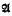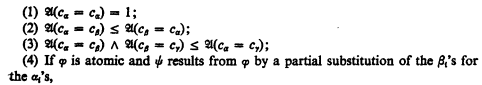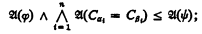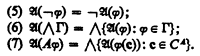Home

# The completeness theorem for infinitary logic

## Extract

It will be proven that a set of sentences of infinitary logic is satisfiable iff it is proof theoretically consistent. Since this theorem is known to be false, it must be quickly added that an extended notion of model is being used; truth values may be taken from an arbitrary complete Boolean algebra. We shall give a Henkin style proof of this result which generalizes easily to Boolean valued sets of sentences.

For each infinite candinal number κ the language Lκ is built up from a set of relation symbols together with a constant symbol cα and a variable υα for each α in κ. It contains atomic formulas and is closed under the following rules:

(1) If Γ is a set of formulas of power < κ ∧ Γ is a set of formulas.

(2) If φ is a formula, ¬ φ is also.

(3) If φ is a formula and A Is a subset of κ of power < κ then is a formula.

∧Γ is meant to be the conjunction of all the formulas in Γ, while is the universal quantification of all the variables υα for α in A. We let C denote the set of constant symbols in Lκ, the parameter κ must be discovered from the context.

A model is identified with its truth function. Thus a model is a functionmapping the sentences of Lκ into a complete Boolean algebra which satisfies the following conditions:Hide All

# The completeness theorem for infinitary logic

## Metrics

### Full text viewsFull text views reflects the number of PDF downloads, PDFs sent to Google Drive, Dropbox and Kindle and HTML full text views.

Total number of HTML views: 0
Total number of PDF views: 0 *Loading metrics...

### Abstract viewsAbstract views reflect the number of visits to the article landing page.

Total abstract views: 0 *Loading metrics...

* Views captured on Cambridge Core between <date>. This data will be updated every 24 hours.

Usage data cannot currently be displayed.